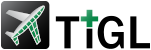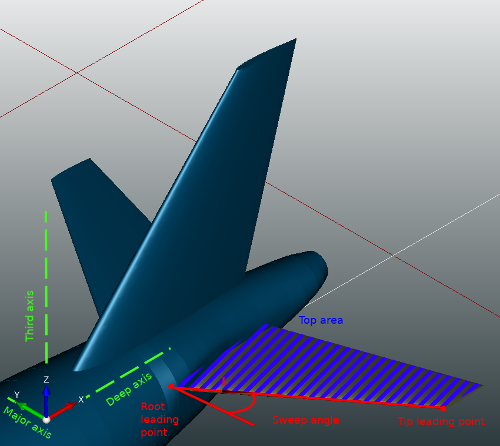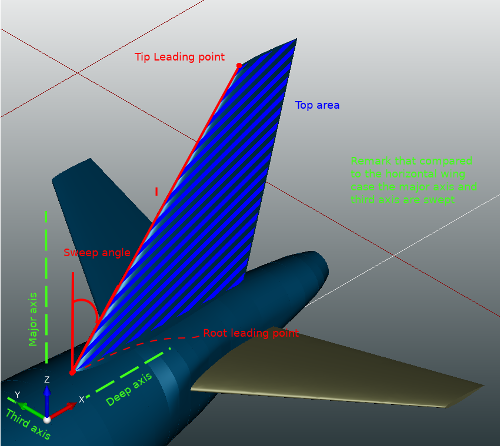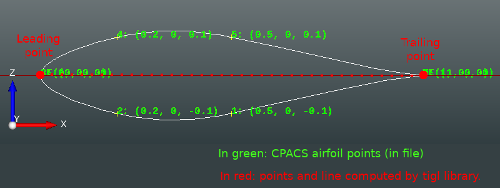3.0.0
CPACSCreator high level parameters

Tigl framework use multiple high level parameter to simplify the edition of CPACS files. In this section, we will present in details the definition used by our functions.

# Wing Parameters Definition

## Sweep angle

Let l be the vector from the root leading point to the tip leading point. Let l' be the projection of l on the plane formed by the major axis and the deep axis. Then, the sweep angle of the wing is the smaller angle between l' and the major axis.Sweep and top area parameter for a horizontal wingSweep and top area parameter for a vertical wing

## Dihedral angle

Let l be the vector from the root leading point to the tip leading point. Let l' be the projection of l on the plane formed by the major axis and the third axis. Then, the dihedral angle of the wing is the smaller angle between l' and the major axis.

## Top area

Let wing' be the projection of the wing on the plane formed by the major axis and the deep axis. Then, the the top area is the area of wing'.

## Span

Let B be a bounding box around the wing where all the edges of the box are parallel either to the major axis, to the deep axis or to the third axis. Then the span is the the size of the box B along the major axis. Remark, that if the wing has a symmetry parameter the "two" wings are present in the box.

## Half span

Let B be a bounding box around the wing where the symmetry parameter of the wing is not applied (if present). Then, the half span is the the size of the box B along the major axis. Remark, in this case always exactly "one" wing is present in the box.

## Aspect ratio

aspect_ratio = 2 * pow(half_span, 2) / top_area

## Major Axis

The major axis is either the X axis, the Y axis or the Z axis of the world coordinate system. The goal of this axis is to define which axis of the world coordinate system is best suited to be use as the span axis. If the wing has a symmetry parameter, we define the major axis has the only axis normal to the symmetry plane. If the wing has no symmetry plane, we use an heuristic. First we define the deep axis as the axis that is more "used" by the chord vector of the wing elements, please see the subsection deep axis for more details. Then, we define the major axis between the two remaining axis as the axis that is more "used" by the leading edges of all segments of the wing. To find out the most "used" axis by the leading edges. Let l be the addition of all leading edges. Then the most "used" axis is the coordinate axis that has the higher values in l. Remark, to make the addition in l, we use the absolute value to capture the delta on this axis.

## Deep Axis

The deep axis is either the X axis, the Y axis or the Z axis of the world coordinate system. The goal of this axis is to define which axis of the world coordinate system is best suited to be used with the major axis to create the plane in which the top area is computed. To define the deep axis, we first exclude the major axis. Then we choose, between the two remaining axis, the axis that is more "used" by the chord vector of the wing elements. To do this, let v be the addition of all the chord vectors, then the most "used" axis is the coordinate axis that has the higher values in v. Remark, to make the addition in v, we use the absolute value to capture the delta on this axis.

## Third Axis

The third axis is either the X axis, the Y axis or the Z axis of the world coordinate system. The goal of this axis is to define which axis of the world coordinate system is best suited to be used to represent the "high" of the wing. To define the third axis, we simply exclude the major axis and the deep axis of the three possibilities.

## Root wing element

The root element is the "from element" of the first segment. Remark, we assume that the segment are order starting form the root in the CPACS file. If it is not the case, tigl library perform a reordering when the file is open.

## Tip wing element

The tip element is defined as the element which its center is most distant from the root element center along the major axis.

## Wing Rotation

The wing rotation is simply defined as the rotation in the wing transformation.

## Trailing point of a wing element

Let p be the trailing point of the associated airfoil and M be the augmented matrix that represent all affine transformations applied on this wing element (element transformation, section transformation, positioning transformation, wing transformation, translation of parent). Then the trailing point te of this wing element is: te = M * p

## Leading point of a wing element

Let p be the leading point of the associated airfoil and M be the augmented matrix that represent the all affine transformations applied on this wing element (element transformation, section transformation, positioning transformation, wing transformation, translation of parent). Then the leading point le of this wing element is: le = M * p

## Chord vector of a wing element

The chord vector is simply the vector that goes form the leading point, le to the trailing point, te.

## Chord point of a wing element

Let v be the chord vector and le be the the leading point of the wing element. Then we define the chord point cp at x percent as: cp(x) = le + x*v Remark, the percentage is given as a value between 0 and 1.

## Center point of a wing element

The center point of an element is defined as the center of mass of the element wire. We name by element wire the associated airfoil after that all affine transformations of the wing are applied on the airfoil profile.

## Trailing point of an airfoil

If the wing airfoil is described as a set of points, the trailing point is simply the first point of the airfoil description. If the wing airfoil is described as function, the trailing edge point definition is not so clear at the moment.Trailing point and leading point of an airfoil described as a set of points

## Leading point of an airfoil

If the wing airfoil is described as a set of points, the leading point is the point of the airfoil description that is the most distant of the airfoil trailing point. If the wing airfoil is described as function, the leading edge point definition is not so clear for the moment.

Coming soon!